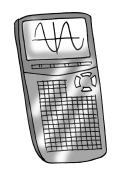### Home > CCAA8 > Chapter 8 Unit 9 > Lesson CCA: 8.1.1 > Problem8-12

8-12.

Calculate the value of each expression below.1.  $5-\sqrt{36}$

• $-1$

1.  $1+\sqrt{39}$

• $\sqrt{39}\approx6.24$

• $1+6.24=7.24$

1.  $-2-\sqrt{5}$